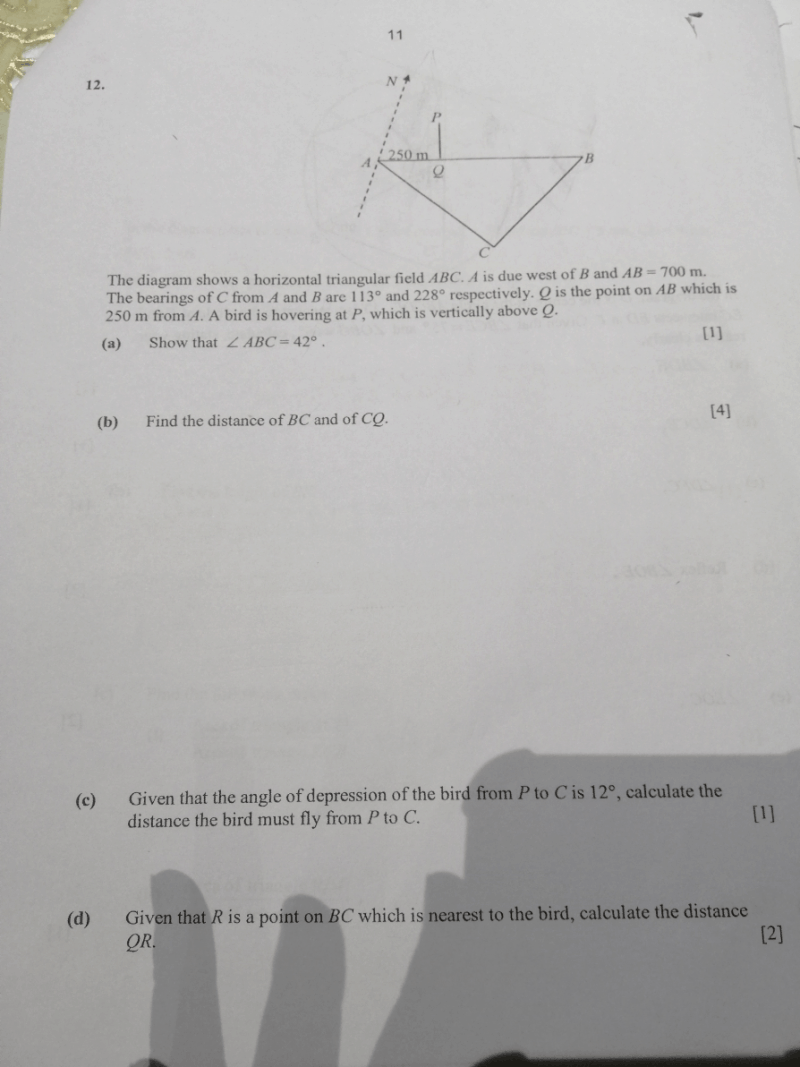# QuestionHow to solve this sec 4 qns.?

1 Answer

# Answer

a) Bearing of C from B: 228°
ABC → 360° – 228° – 90° (anti-clockwise turn from B to C)
= 42
°

b) Bearing of C from A: 113°
∠BAC → 113° – 90° = 23°

Using Law of Sines,

sin 115 ÷ AB = sin 23 ÷ BC
sin 115 ÷ 700 = sin 23 ÷ BC
BC = 700 sin 23 ÷ sin 115
301.79 m

Using Law of Cosines,

(QC)2 = (BQ)2 + (BC)2 – 2(BQ)(BC) x cos (ABC)
(QC)2 = 4502 + 301.792 – 2(450)(301.79) x c0s 42
QC = sqrt[4502 + 301.792 – 2(450)(301.79) x c0s 42]
302.87 m

c) △PQC is a right triangle

sin 12 = 302.87 ÷ PC
PC = 302.78 ÷ sin 12
1456.29 m

d) QR is shortest distance from P to a point on BC, ∴ QR must be height of △BQC (with BC as base)

With reference to △BQR,

sin 42 = QR ÷ 450
QR = sin 42 x 450
301.11 m

0 Replies 0 Likes ✔Accepted Answer